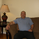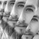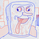TradingView17210 lượt xem
This is an experimental study which calculates a linear regression channel over a specified period or interval using custom moving average types for its calculations.

Linear regression is a linear approach to modeling the relationship between a dependent variable and one or more independent variables.
In linear regression , the relationships are modeled using linear predictor functions whose unknown model parameters are estimated from the data.

The regression channel in this study is modeled using the least squares approach with four base average types to choose from:
-> Arnaud Legoux Moving Average ( ALMA )
-> Exponential Moving Average ( EMA )
-> Simple Moving Average ( SMA )
-> Volume Weighted Moving Average ( VWMA )
When using VWMA , if no volume is present, the calculation will automatically switch to tick volume , making it compatible with any cryptocurrency, stock, currency pair, or index you want to analyze.

There are two window types for calculation in this script as well:
-> Continuous, which generates a regression model over a fixed number of bars continuously.
-> Interval, which generates a regression model that only moves its starting point when a new interval starts. The number of bars for calculation cumulatively increases until the end of the interval.

The channel is generated by calculating standard deviation multiplied by the channel width coefficient, adding it to and subtracting it from the regression line, then dividing it into quartiles.

To observe the path of the regression, I've included a tracer line, which follows the current point of the regression line. This is also referred to as a Least Squares Moving Average ( LSMA ).

For added predictive capability, there is an option to extend the channel lines into the future.

A custom bar color scheme based on channel direction and price proximity to the current regression value is included.

I don't necessarily recommend using this tool as a standalone, but rather as a supplement to your analysis systems.
Regression analysis is far from an exact science. However, with the right combination of tools and strategies in place, it can greatly enhance your analysis and trading.
Phát hành các Ghi chú: Updates:
-> Script structure has been reorganized.
-> Corrected NaN filter values in initial states.
-> Added Rolling Moving Average and Linear Weighted Moving Average to the list of available base averages.
-> Added style customization for the channel lines. Because TV doesn't have built-in style inputs for line objects, you'll find these settings in the "Inputs" section. For each channel line, you can now adjust:
- If the line is drawn - selected via the specified toggle switch
- Width of the line - can be any integer greater than zero
- Style of the line - available styles are solid, dotted, and dashed
- Color of the line - uses my RGB color editor, which has 216 colors to choose from via the specified R, G, and B inputs
Mã nguồn mở

Với tinh thần của TradingView, tác giả đã xuất bản tập lệnh theo mã nguồn mở, vì thế trader có thể dễ dàng hiểu và tùy chỉnh được. Bạn có thể sử dụng miễn phí, hoặc tùy chỉnh lại mã đã được cấp phép bởi Quy tắc Chung. Bạn có thể sử dụng nó trên biểu đồ.

Bạn muốn sử dụng tập lệnh này trên biểu đồ?
For my full list of premium tools, check the blog:
https://wallanalytics.com/

Reach out on Telegram:
https://t.me/DonovanWall

## Bình luậnWhat three parameters we are expecting here?

//Correlation Coefficient
corr(x, y, t)=>

Close, length and something else?

Thanks.
Phản hồisyracusepro
@syracusepro, Great question. x is the bar number counting upward, y is the price value, and t is the sampling length. The slope parameter b is dependent on correlation between price and time.
Phản hồiThank you for the new script once again. I'm a big fan!
Phản hồi